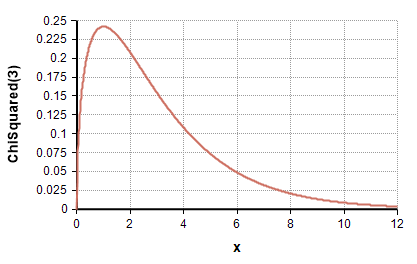# Chi-squared distribution

(Redirected from ChiSquared)

Release: 4.6  •  5.0  •  5.1  •  5.2  •  5.3  •  5.4  •  6.0  •  6.1  •  6.2  •  6.3  •  6.4

The $\displaystyle{ \Chi^2 }$-squared distribution is a continuous, positive only, unimodal probability distribution that describes the sum of independent normally-distributed random variables.The Chi-squared distribution with «dof» degrees of freedom describes the distribution of a Chi-Squared metric defined as

$\displaystyle{ \Chi^2 \sum_{i=1}^n {y_i}^2 }$

where each yi is independently sampled from a standard normal distribution and «dof» = n - 1 . The distribution is defined over nonnegative values.

The Chi-squared distribution is commonly used for analyses of second moments, such as analyses of variance and contingency table analyses. The ratio of two chi-squared-distributed variables follows an F-distribution.

## Functions

### ChiSquared(dof)

The distribution function. Use this to define a chance variable or other uncertain quantity with an F-distribution with «dof» degrees of freedom.

### DensChiSquared(x, dof)

The analytic probability density at «x». Equal to

$\displaystyle{ p(x) = {1\over{2^{d/2} \Gamma(d/2)}} x^{d/2-1} e^{-x/2} }$

where $\displaystyle{ d }$ is «dof» and $\displaystyle{ \Gamma(x) }$ is the GammaFn(x).

### CumChiSquared(x, dof)

The analytic cumulative density up to «x». This is the probability that a random sample will be less than or equal to «x».

### CumChiSquaredInv(p, dof)

The inverse cumulative density (quantile function), which computes the pth fractile/quantile/percentile value x, which has a «p» probability of being greater than or equal to a random variate draw from a chi-squared distribution with «dof» degrees of freedom.

## Statistics

Theoretical statistics (i.e., in the absence of sampling error) are:

## Examples

Suppose

Variable V := ChiSquared(k)
Variable W := ChiSquared(m)
Variable S := (V/k)*(W/m)

S is distributed as an F-distribution with k and m degrees of freedom.

The F distribution is useful for the analysis of ratios of variance, such as a one-factor between-subjects analysis of variance.